Last updated: 2017-02-17

Code version: 0a96ec4f599f56f6968bec3929589cf0212b40a9

# Caveat on code

Note: the code here is designed to be readable by a beginner, rather than “efficient”. The idea is that you can use this code to learn about the basics of MCMC, but not as a model for how to program well in R!

# Example 1: sampling from an exponential distribution using MCMC

Any MCMC scheme aims to produce (dependent) samples from a target" distribution. In this case we are going to use the exponential distribution with mean 1 as our target distribution. So we start by defining our target density:

target = function(x){
if(x<0){
return(0)}
else {
return( exp(-x))
}
}

Having defined the function, we can now use it to compute a couple of values (just to illustrate the idea of a function):

target(1)
 0.3678794
target(-1)
 0

Next, we will program a Metropolis–Hastings scheme to sample from a distribution proportional to the target

x = rep(0,1000)
x = 3     #this is just a starting value, which I've set arbitrarily to 3
for(i in 2:1000){
currentx = x[i-1]
proposedx = currentx + rnorm(1,mean=0,sd=1)
A = target(proposedx)/target(currentx)
if(runif(1)<A){
x[i] = proposedx       # accept move with probabily min(1,A)
} else {
x[i] = currentx        # otherwise "reject" move, and stay where we are
}
}

Note that x is a realisation of a Markov Chain. We can make a few plots of x:

plot(x)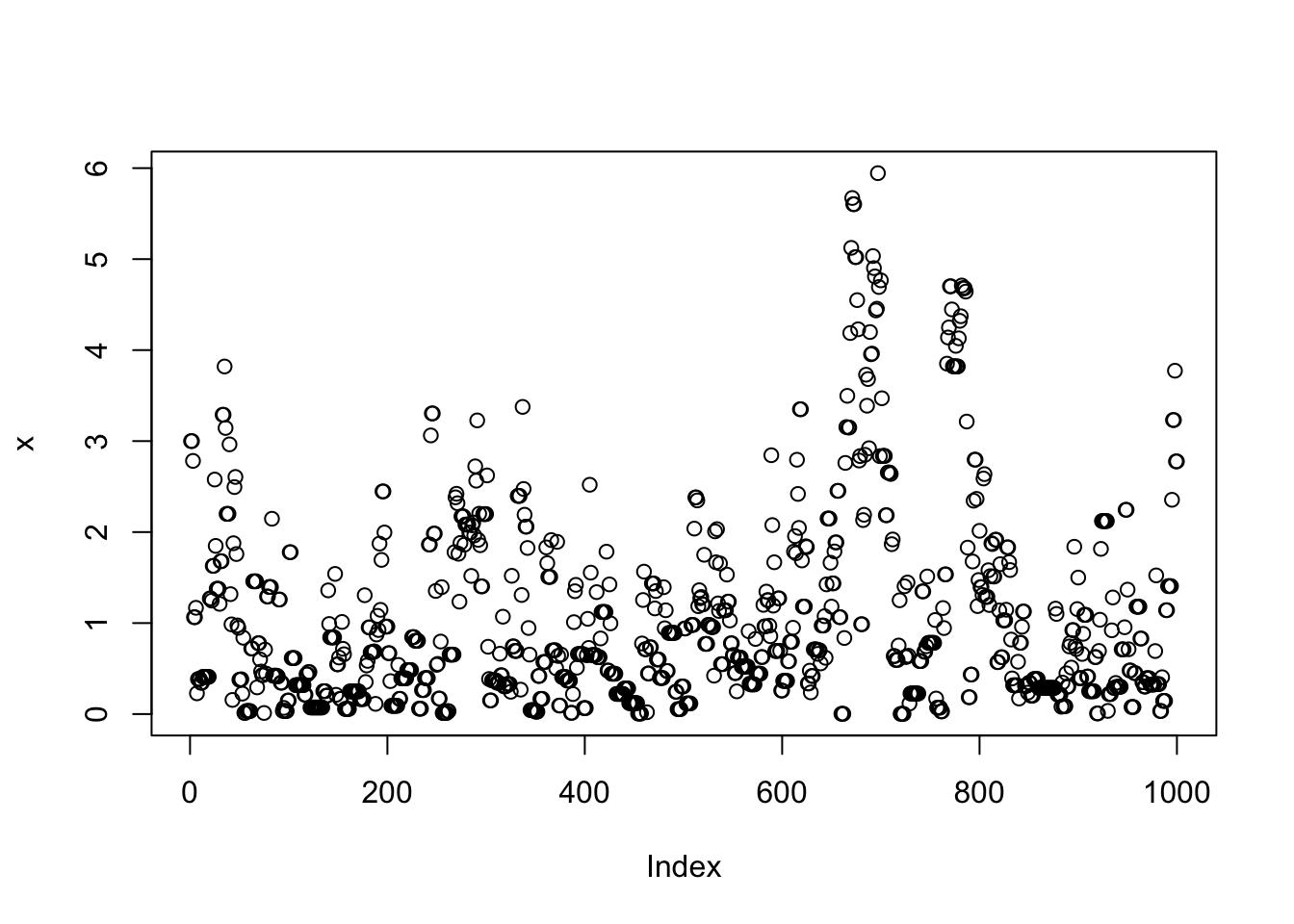hist(x)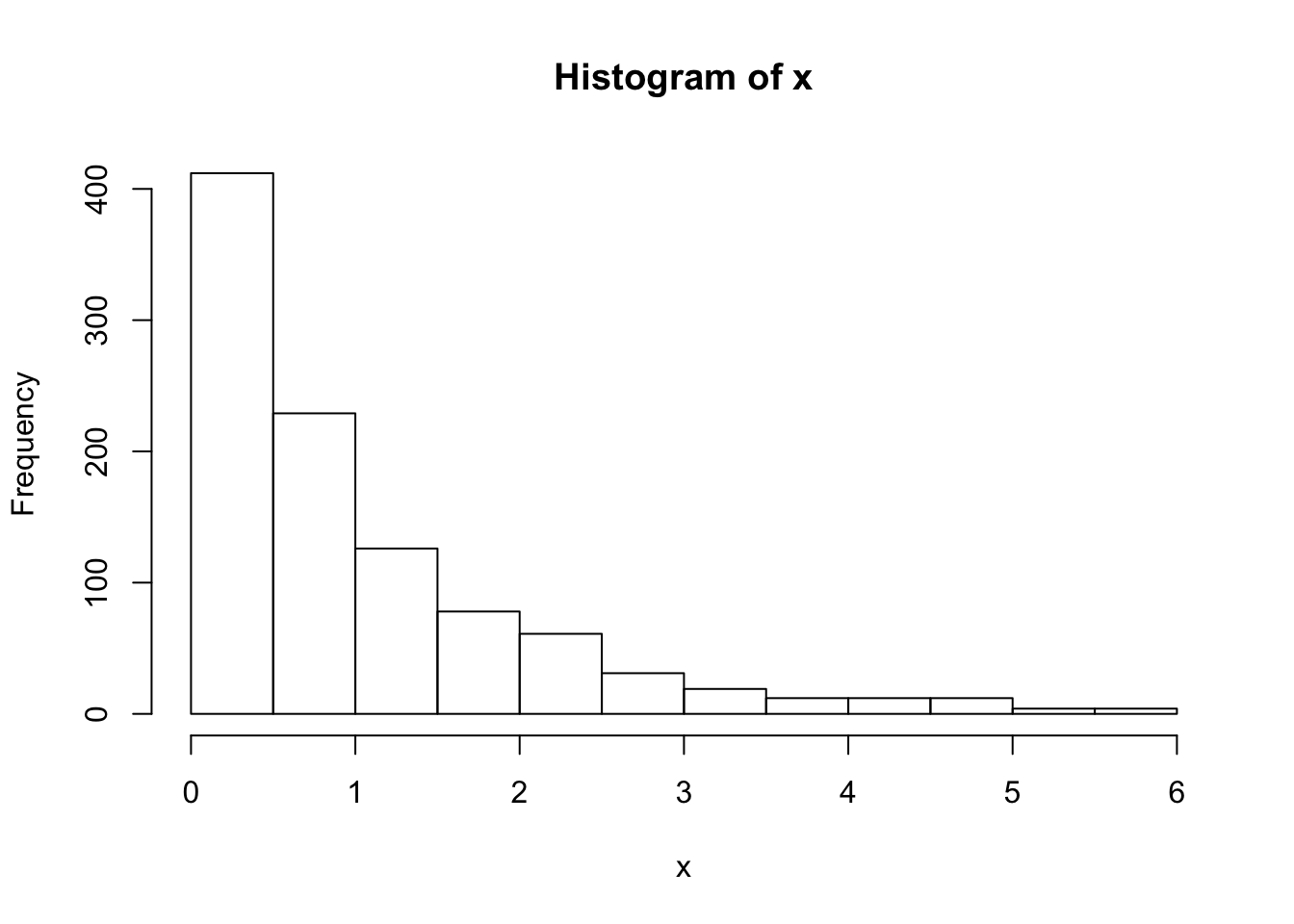We can wrap this up in a function to make things a bit neater, and make it easy to try changing starting values and proposal distributions

easyMCMC = function(niter, startval, proposalsd){
x = rep(0,niter)
x = startval
for(i in 2:niter){
currentx = x[i-1]
proposedx = rnorm(1,mean=currentx,sd=proposalsd)
A = target(proposedx)/target(currentx)
if(runif(1)<A){
x[i] = proposedx       # accept move with probabily min(1,A)
} else {
x[i] = currentx        # otherwise "reject" move, and stay where we are
}
}
return(x)
}

Now we’ll run the MCMC scheme 3 times, and look to see how similar the results are:

z1=easyMCMC(1000,3,1)
z2=easyMCMC(1000,3,1)
z3=easyMCMC(1000,3,1)

plot(z1,type="l")
lines(z2,col=2)
lines(z3,col=3)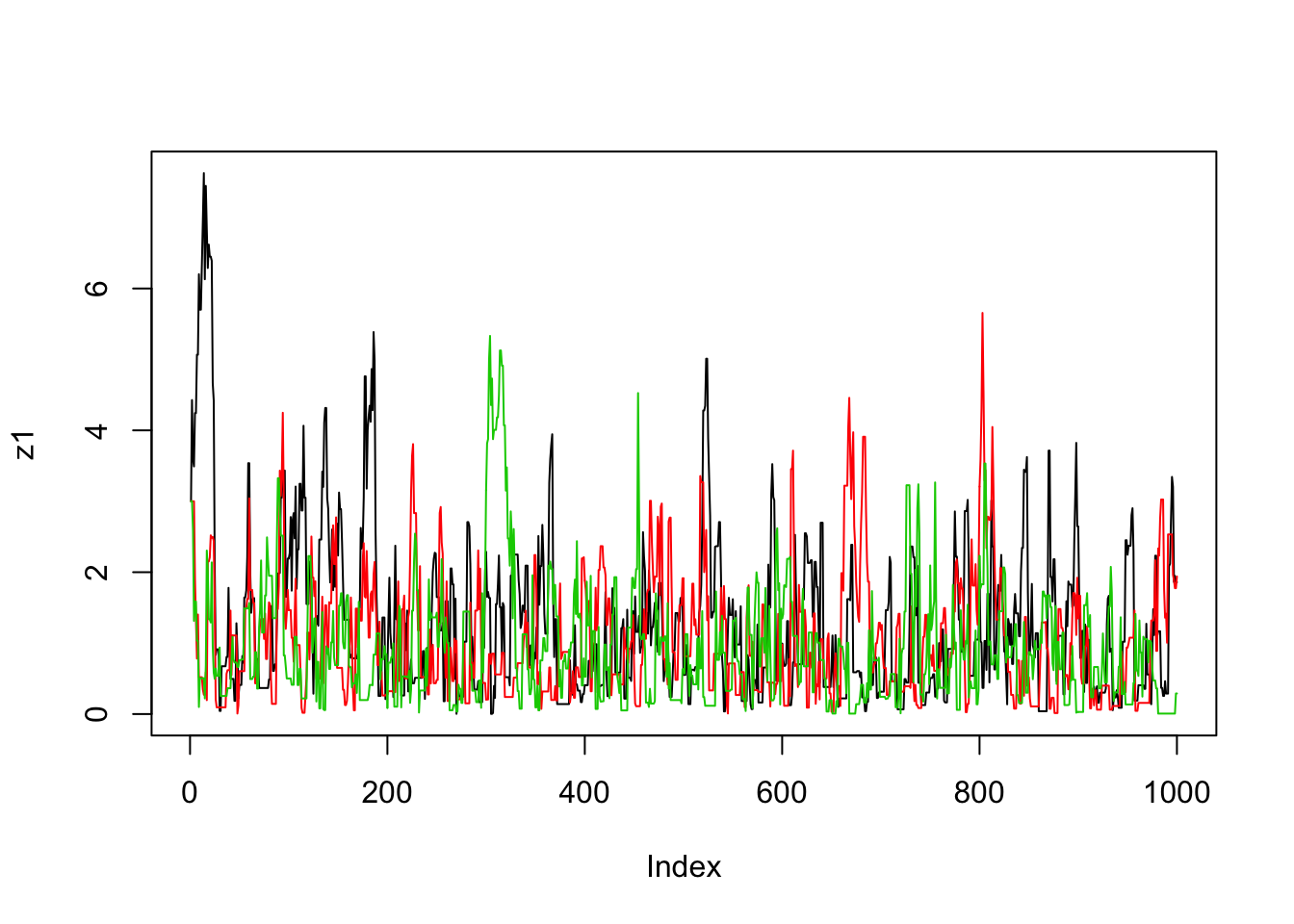par(mfcol=c(3,1)) #rather odd command tells R to put 3 graphs on a single page
maxz=max(c(z1,z2,z3))
hist(z1,breaks=seq(0,maxz,length=20))
hist(z2,breaks=seq(0,maxz,length=20))
hist(z3,breaks=seq(0,maxz,length=20))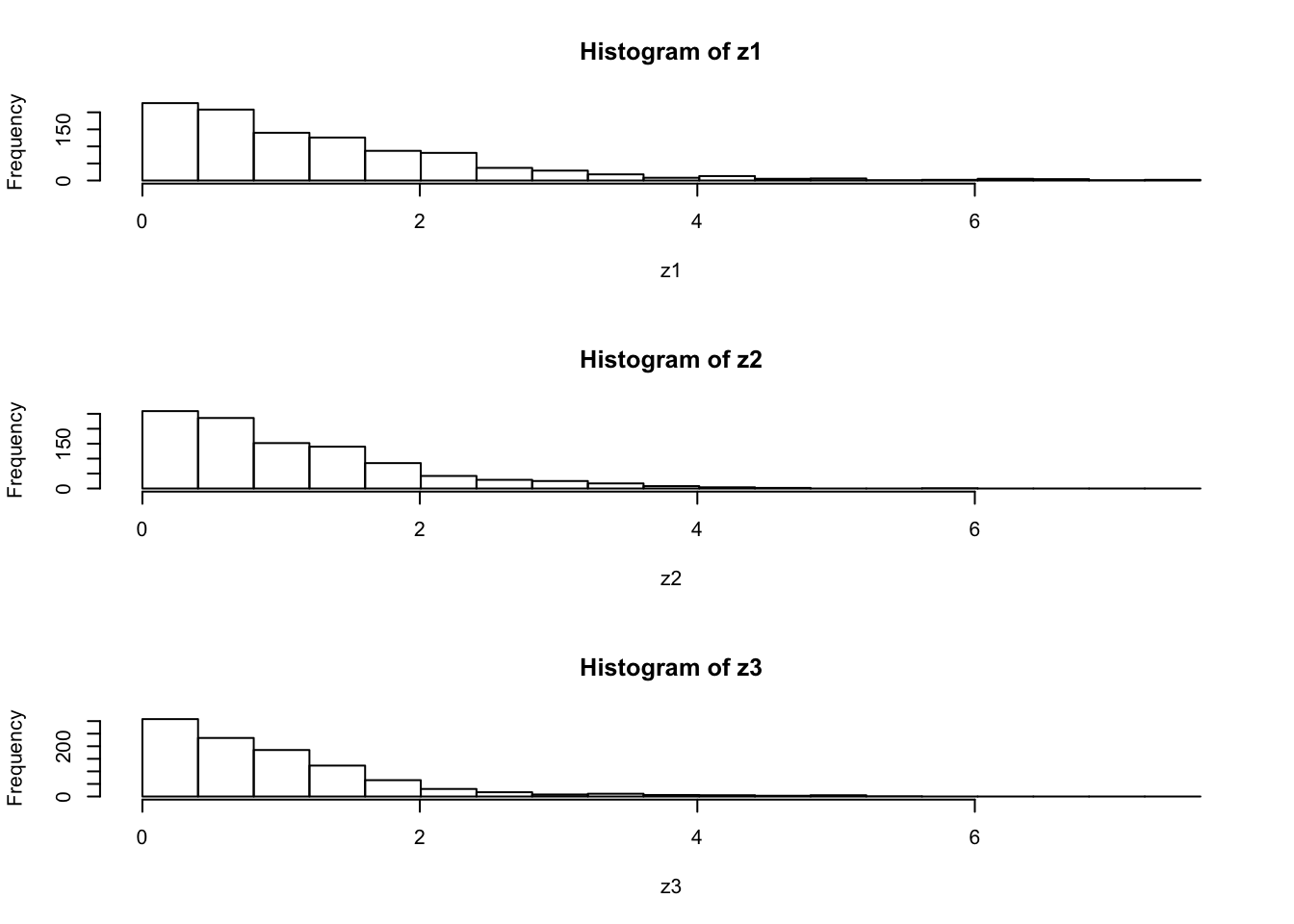## Exercise

Use the function easyMCMC to explore the following:

1. how do different starting values affect the MCMC scheme?
2. what is the effect of having a bigger/smaller proposal standard deviation?
3. try changing the target function to the following
target = function(x){

return((x>0 & x <1) + (x>2 & x<3))
}

What does this target look like? What happens if the proposal sd is too small here? (try eg 1 and 0.1)

# Example 2: Estimating an allele frequency

A standard assumption when modelling genotypes of bi-allelic loci (eg loci with alleles $$A$$ and $$a$$) is that the population is “randomly mating”. From this assumption it follows that the population will be in “Hardy Weinberg Equilibrium” (HWE), which means that if $$p$$ is the frequency of the allele $$A$$ then the genotypes $$AA$$, $$Aa$$ and $$aa$$ will have frequencies $$p^2, 2p(1-p)$$ and $$(1-p)^2$$ respectively.

A simple prior for $$p$$ is to assume it is uniform on $$[0,1]$$. Suppose that we sample $$n$$ individuals, and observe $$n_{AA}$$ with genotype $$AA$$, $$n_{Aa}$$ with genotype $$Aa$$ and $$n_{aa}$$ with genotype $$aa$$.

The following R code gives a short MCMC routine to sample from the posterior distribution of $$p$$. Try to go through the code to see how it works.

prior = function(p){
if((p<0) || (p>1)){  # || here means "or"
return(0)}
else{
return(1)}
}

likelihood = function(p, nAA, nAa, naa){
return(p^(2*nAA) * (2*p*(1-p))^nAa * (1-p)^(2*naa))
}

psampler = function(nAA, nAa, naa, niter, pstartval, pproposalsd){
p = rep(0,niter)
p = pstartval
for(i in 2:niter){
currentp = p[i-1]
newp = currentp + rnorm(1,0,pproposalsd)
A = prior(newp)*likelihood(newp,nAA,nAa,naa)/(prior(currentp) * likelihood(currentp,nAA,nAa,naa))
if(runif(1)<A){
p[i] = newp       # accept move with probabily min(1,A)
} else {
p[i] = currentp        # otherwise "reject" move, and stay where we are
}
}
return(p)
}

Running this sample for $$n_{AA}$$ = 50, $$n_{Aa}$$ = 21, $$n_{aa}$$=29.

z=psampler(50,21,29,10000,0.5,0.01)

Now some R code to compare the sample from the posterior with the theoretical posterior (which in this case is available analytically; since we observed 121 $$A$$s, and 79 $$a$$s, out of 200, the posterior for $$p$$ is Beta(121+1,79+1).

x=seq(0,1,length=1000)
hist(z,prob=T)
lines(x,dbeta(x,122, 80))  # overlays beta density on histogram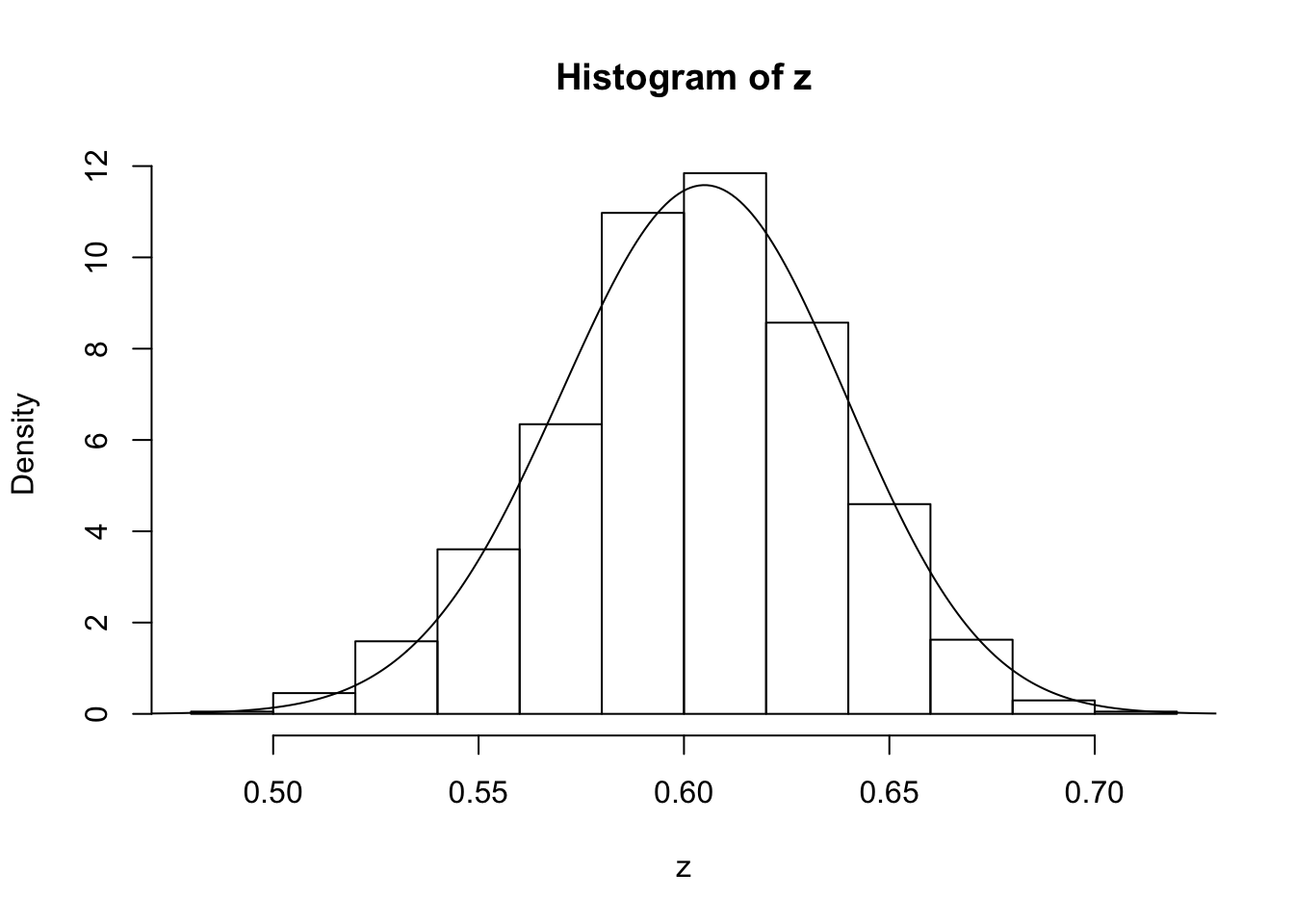You might also like to discard the first 5000 z’s as “burnin”. Here’s one way in R to select only the last 5000 zs

hist(z[5001:10000])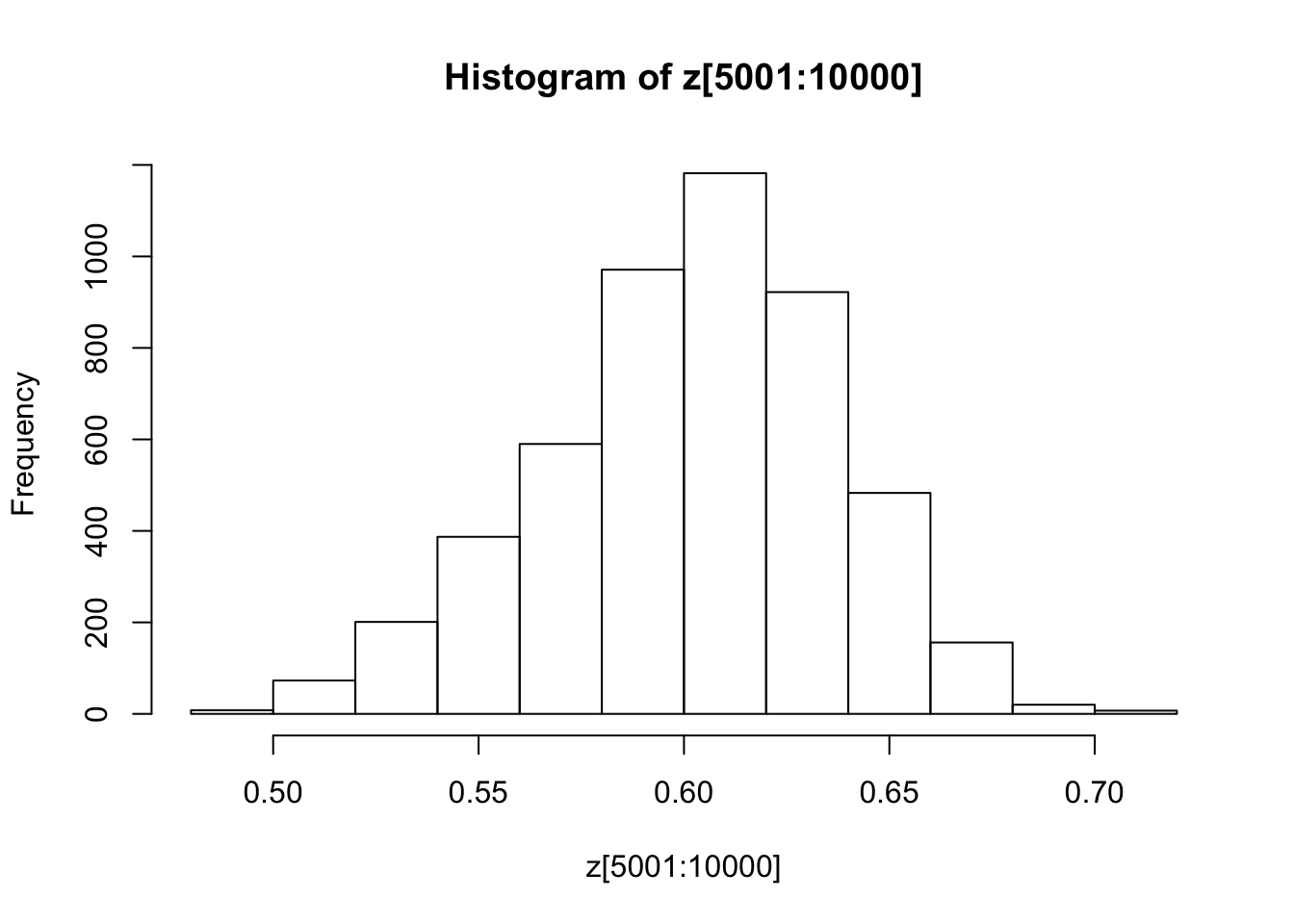## Exercise

Investigate how the starting point and proposal standard deviation affect the convergence of the algorithm.

# Example 3: Estimating an allele frequency and inbreeding coefficient

A slightly more complex alternative than HWE is to assume that there is a tendency for people to mate with others who are slightly more closely-related than “random” (as might happen in a geographically-structured population, for example). This will result in an excess of homozygotes compared with HWE. A simple way to capture this is to introduce an extra parameter, the “inbreeding coefficient” $$f$$, and assume that the genotypes $$AA$$, $$Aa$$ and $$aa$$ have frequencies $$fp + (1-f)p*p, (1-f) 2p(1-p)$$, and $$f(1-p) + (1-f)(1-p)(1-p)$$.

In most cases it would be natural to treat $$f$$ as a feature of the population, and therefore assume $$f$$ is constant across loci. For simplicity we will consider just a single locus.

Note that both $$f$$ and $$p$$ are constrained to lie between 0 and 1 (inclusive). A simple prior for each of these two parameters is to assume that they are independent, uniform on $$[0,1]$$. Suppose that we sample $$n$$ individuals, and observe $$n_{AA}$$ with genotype $$AA$$, $$n_{Aa}$$ with genotype $$Aa$$ and $$n_{aa}$$ with genotype $$aa$$.

## Exercise:

• Write a short MCMC routine to sample from the joint distribution of $$f$$ and $$p$$.

Hint: here is a start; you’ll need to fill in the …

fpsampler = function(nAA, nAa, naa, niter, fstartval, pstartval, fproposalsd, pproposalsd){
f = rep(0,niter)
p = rep(0,niter)
f = fstartval
p = pstartval
for(i in 2:niter){
currentf = f[i-1]
currentp = p[i-1]
newf = currentf + ...
newp = currentp + ...
...
}
return(list(f=f,p=p)) # return a "list" with two elements named f and p
}
• Use this sample to obtain point estimates for $$f$$ and $$p$$ (e.g. using posterior means) and interval estimates for both $$f$$ and $$p$$ (e.g. 90% posterior credible intervals), when the data are $$n_{AA} = 50, n_{Aa} = 21, n_{aa}=29$$.

You could also tackle this problem with a Gibbs Sampler (see vignettes here and here).

To do so you will want to use the following “latent variable” representation of the model: $z_i \sim Bernoulli(f)$ $p(g_i=AA | z_i=1) = p; p(g_i=AA | z_i=0) = p^2$ $p(g_i=Aa | z_i = 1)= 0; p(g_i=Aa | z_i=0) = 2p(1-p)$ $p(g_i=aa | z_i = 1) = (1-p); p(g_i =aa | z_i=0) = (1-p)^2$

Summing over $$z_i$$ gives the same model as above: $p(g_i=AA) = fp + (1-f)p^2$

## Exercise:

Unsing the above, implement a Gibbs Sampler to sample from the joint distribution of $$z,f,$$ and $$p$$ given genotype data $$g$$.

Hint: this requires iterating the following steps

1. sample $$z$$ from $$p(z | g, f, p)$$
2. sample $$f,p$$ from $$p(f, p | g, z)$$

## Session Information

sessionInfo()
R version 3.3.2 (2016-10-31)
Platform: x86_64-apple-darwin13.4.0 (64-bit)
Running under: OS X El Capitan 10.11.6

locale:
 en_US.UTF-8/en_US.UTF-8/en_US.UTF-8/C/en_US.UTF-8/en_US.UTF-8

attached base packages:
 stats     graphics  grDevices utils     datasets  methods   base

other attached packages:
 workflowr_0.3.0 rmarkdown_1.3   ggplot2_2.1.0   dplyr_0.5.0
 magrittr_1.5    shiny_1.0.0     mixfdr_1.0      ashr_2.1.2
 dscr_0.1.1      horseshoe_0.1.0

loaded via a namespace (and not attached):
 Rcpp_0.12.9        git2r_0.18.0       plyr_1.8.4
 iterators_1.0.8    tools_3.3.2        digest_0.6.10
 memoise_1.0.0      tibble_1.2         jsonlite_1.2
 evaluate_0.10      gtable_0.2.0       lattice_0.20-34
 Matrix_1.2-7.1     foreach_1.4.3      DBI_0.5-1
 rstudioapi_0.6     curl_2.2           yaml_2.1.14
 parallel_3.3.2     httr_1.2.1         withr_1.0.2
 stringr_1.1.0      knitr_1.15.1       devtools_1.12.0
 REBayes_0.73       rprojroot_1.2      grid_3.3.2
 R6_2.2.0           EbayesThresh_1.3.2 reshape2_1.4.2
 whisker_0.3-2      backports_1.0.5    scales_0.4.0
 codetools_0.2-15   htmltools_0.3.5    MASS_7.3-45
 assertthat_0.1     mime_0.5           colorspace_1.2-7
 xtable_1.8-2       httpuv_1.3.3       labeling_0.3
 stringi_1.1.2      Rmosek_7.1.2       lazyeval_0.2.0
 munsell_0.4.3      doParallel_1.0.10  pscl_1.4.9
 truncnorm_1.0-7    SQUAREM_2016.8-2  

This site was created with R Markdown